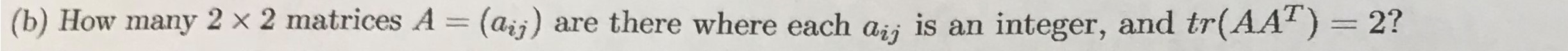# (b) How many 2 × 2 matrices A = (aij) are there where each ai; is an integer, and tr(AAT) = 2?%3D%3D

Question
100 views

The qestion in the picture is a linear algebra/differential equations question:help_outlineImage Transcriptionclose(b) How many 2 × 2 matrices A = (aij) are there where each ai; is an integer, and tr(AAT) = 2? %3D %3D fullscreen
check_circle

Step 1

Given

Step 2

Find the number of matrices such that

Step 3

### Want to see the full answer?

See Solution

#### Want to see this answer and more?

Solutions are written by subject experts who are available 24/7. Questions are typically answered within 1 hour.*

See Solution
*Response times may vary by subject and question.
Tagged in

### Math